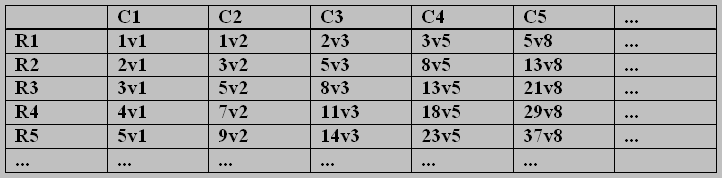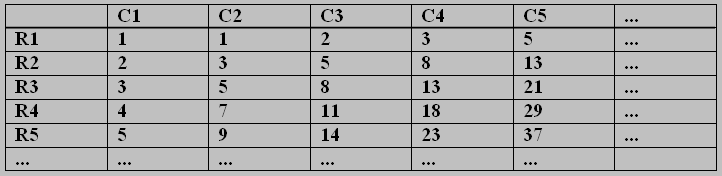## CNB7 Enteger Sequences, and a curious AP/FP Pattern Matrix

August 25th, 2012

Good Morning!

This Blog seems to diverge from the topic of  Cycle Numbers. True, but not very far. It will give readers a chance to play mentally with entegers (a simple notion introduced by me in, for example, Schaake, A. G. and Turner, J. C. “The Elements of Enteger Geometry”, Applications of Fibonacci Numbers, Vol. 5, Kluwer A. P., 1993, pp. 569 — 583;  and to puzzle over a Cantorian paradox. I hope you can resolve the paradox for me.

On Enteger Sequences

Given an enteger svt, where s and t are integers. (This should be written as s sub t, but I haven’t learned how to include subscripts using WordPress! An ‘enteger’  just means an ordered pair of integers, arranged on a forward diagonal.)  Addition of two entegers is done as with two vectors. An example enteger is  e = 2v3 (i.e. 2 sub 3) : and an example addition is  2v3 + 3v5 = 5v8 .

We can produce enteger Arithmetic Progressions AP(e), and Fibonacci Progressions FP(e) by use of the following operators:

1. Operator A(svt) ≡ (s+t)v(t) , which produces an enteger AP when applied successively,
2. Operator F(svt) ≡ (t)v(s+t) , which produces an enteger FP when applied successively.

N.B. I think there is a pleasing commutative symmetry in this pair of operators.

An enteger Matrix in E (say M(E))

(Recall that E is the enteger matrix which maps to T , using the kappa function.)

We use the operators A on matrix columns, and B on matrix rows, to produce a doubly-infinite matrix M(E) thus:The AP/FP Matrix of Entegers (say M(E))

Note that all the column sequences are so-called double-Arithmetic Progressions; whilst all the row aequences are so-called double-Fibonacci Progressions. All these have been obtained by successive operations of A and F respectively, beginning with enteger 1v1.

It is worthy of note that every pair s,t in all cells of M is coprime.  Applying kappa to all pairs changes M into a unit matrix (a U-matrix, with all elements equal to 1).

The next matrix shows the result of removing all the t-values from the entegers, leaving only the integer s-values:The AP/FP Matrix of Integers (say M(I))

The column APs and the row FPs can now be seen clearly as integer sequences, heading off to infinity in the y- and x-directions respectively.

A Curious Paradox on Transfinite Counts

We end this Blog by describing a paradoxical event,  involving counting the number of prime integers in the second matrix. Clearly there are repetitions involved: even in the small table we have shown, there are two 1s, two 2s, three 3s, and four 5s, four 8s etc. (query:  are only Fibonacci numbers repeated?)

It is long known (Dirichlet, 1837) that every A.P.  starting with coprime first and second elements has an inifinity of primes. Whereas it is an open* question whether or not any Fibomacci sequence has an infinity of primes.

*(Note:  Paul Bruckman and I have posted a paper on this very website, claiming to prove that every Fibonacci sequence with coprime starting pair has an infinity of primes; but for over three years the paper has languished in Mathematics Journal Editors’ pending boxes — so far it hasn’t been accepted for publication, although no-one has pointed out any invalid step in the proofs.)

My Paradox, drawn from the matrix, is this:

Suppose I make the hypothesis that ‘no Fibonacci Progression has an infinity of primes’.  Let me call this the Fib. Prog. Primes Conjecture, or FPPC.

Assume that the FPPC is true.   Then:

(i)  Every row in matrix M(I) has a finite number of primes in it;

(ii) Every column in M(I), being an A.P. with coprime starting pair,  has an infinite number of primes (Dirichlet).

Counting the primes from the rows, taken row-by-row, leads to a matching with the list of natural numbers; therefore their total is the first transfinite number  ω,  since the number of rows tends to infinity.

Counting the primes from the columns, each of which posesses an infinity of them, leads to a total of a higher order of infinity (surely so if we consider ordinalities).

This would seem to be an impossibility, since we are counting a multi-set of primes, arranged in a matrix, and counting them  in two different ways.

An alternative viewpoint would be to map all of the primes registered from the Fibonacci rows into the single column C1, an A.P., which contains the countably infinite set of the primes. That leaves all primes in the remaining infinite sequence of columns C2, C3, C4, …, each having an infinity of primes, unmapped by primes from the Fibonacci rows.

I would be grateful if some reader with better knowledge of Cantor’s arithmetic of transfinite numbers would explain to me this seeming paradox.

If, on the other hand, it is not a paradox, then it has demonstrated the falsity of our assumption of the truth of the FPPC. This would mean that at least one Fibonacci sequence has an infinity of primes.  And I believe that from there we can prove that ALL such Fibonacci progressions have an infinity of primes.

End of Blog.